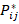Chapter 16.7, Problem 1E

Chapter
Section
Textbook Problem

Let S be the surface of the box enclosed by the planes x = ±1, y = ±1, z = ±1. Approximate ∬S cos(x + 2y + 3z) dS by using a Riemann sum as in Definition 1, taking the patches Sij to be the squares that are the faces of the box S and the pointsto be the centers of the squares.

To determine

To find: The approximate value of Scos(x+2y+3z)dS .

Explanation

Given data:

x=±1,y=±1 and z=±1 , that is the centers of the faces are (±1,0,0),(0,±1,0) and (0,0,±1) .

The box is a cube and the surface area has 4.

Find Scos(x+2y+3z)dS .

Scos(x+2y+3z)dS{[cos(1+2(0)+3(0))](4)+[cos(1+2(0)+3(0))](4)+[cos(0+2(1)+3(0))](4)+[cos(0+2(1)+3(0))](4)+[cos(0+2(0)+3(1))](4)+[cos(0+2(0)+3(1))](<

Still sussing out bartleby?

Check out a sample textbook solution.

See a sample solution

The Solution to Your Study Problems

Bartleby provides explanations to thousands of textbook problems written by our experts, many with advanced degrees!

Get Started

Solve the equations in Exercises 126. (x2+1)x+1(x+1)3=0

Finite Mathematics and Applied Calculus (MindTap Course List)

Let and g(x) = x + 3. Then (g ∘ h)(x) = ______.

Study Guide for Stewart's Single Variable Calculus: Early Transcendentals, 8th

The length of the curve given by x = 3t2 + 2, y = 2t3, is:

Study Guide for Stewart's Multivariable Calculus, 8th

In Exercises 710, find C(n,x)pxqnx for the given values of n, x and p. n=6,x=5,p=.4

Finite Mathematics for the Managerial, Life, and Social Sciences